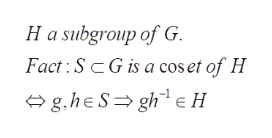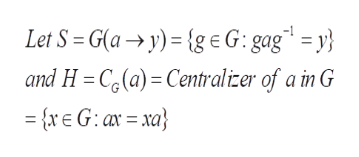# 7Suppose that a E G. For each y in the conjugacy class of a, let G(a y)= {g E G: gag= y}be the set of group elements which conjugate a into y. Prove that G(a y) is a coset of CG(a)1

Question
1 viewshelp_outlineImage Transcriptionclose7 Suppose that a E G. For each y in the conjugacy class of a, let G(a y)= {g E G: gag= y} be the set of group elements which conjugate a into y. Prove that G(a y) is a coset of CG(a) 1 fullscreen
check_circle

Step 1

To prove that the given subset G(a -> y) is a coset of the centralizer of a in G.

Step 2

Recall the general fact about subgroups and cosets in any group Ghelp_outlineImage TranscriptioncloseH a subgroup of G Fact SG is a cos et of H g,heSgheH fullscreen
Step 3

Apply this criterion to subset S and ...help_outlineImage TranscriptioncloseLet S = G(a y) {g eG: gag = y} and H =C(a) Centralicer of a in G =r G: axa fullscreen

### Want to see the full answer?

See Solution

#### Want to see this answer and more?

Solutions are written by subject experts who are available 24/7. Questions are typically answered within 1 hour.*

See Solution
*Response times may vary by subject and question.
Tagged in

### Math